# User:IssaRice/Distribution of X over Y

A distribution of$X$ over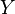$Y$ is any function$f : Y \to X$.

Examples:

• A probability distribution over a finite sample space is a distribution of probabilities$[0,1]$ over the sample space$\Omega$. In other words, a probability distribution is a function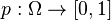$p : \Omega \to [0,1]$. In the case of probability distributions, we also require some extra conditions (namely that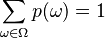$\sum_{\omega \in \Omega} p(\omega) = 1$).
• A wealth distribution over people is a distribution of wealth ($\mathbf R$) over a set of people ($P$), that is, a function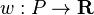$w : P \to \mathbf R$, where$P$ is a set of people. Thus, if$x \in P$ then$w(x)$ is$x$'s wealth.
• A gender distribution over people assigns a gender to each person.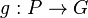$g : P \to G$
• A gender distribution can also be thought of as a distribution of sets of people over genders.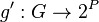$g' : G \to 2^P$ where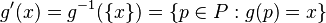$g'(x) = g^{-1}(\{x\}) = \{p \in P : g(p) = x\}$
• A random variable is a distribution of real values over a sample space.
• A frequency distribution of test scores is a distribution of frequencies over test scores, i.e. a function$f : \mathbf R \to \mathbf N$ (where test scores are real and the frequency is a natural number)

The definition above runs into trouble when the set$Y$ is uncountable. In this case, we might not be able to find any function that satisfies the extra conditions we want to place on the distribution (e.g. in the case of probability distributions, we want to assign 0 to every individual outcome, but then the sum is also 0 rather than 1).

It seems like one way to get around this is to change the type of a distribution. Namely, rather than a function$f : Y \to X$ we have some collection$\mathcal F \subseteq 2^Y$ of subsets of$Y$, and we define a function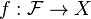$f : \mathcal F \to X$. What constraints should this new function satisfy? In the case of probability, we have a condition that translates between subsets and real numbers. In general, we need some notion of a "merge": if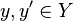$y,y' \in Y$ have "stuff"$x,x' \in X$ respectively, then how much "stuff" in$X$ does the "merged"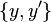$\{y,y'\}$ have? In the case of probabilities and wealth (and other things that take real numbers) we can add, but it seems like we can't ask what the "combined" gender of two people is.

The other way to generalize to uncountable sets seems to be to look at density (like a probability density function). But it seems like we can get density via the subsets method above.

Can we restrict$X$ to be finite, then define$f : \mathcal F \to \mathbf R^{X}$? Then we can force the outcome to be a vector. In the case of gender we have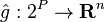$\hat g : 2^P \to \mathbf R^n$, and$\hat g(S) := \sum_{p\in S} g(p)$, so it's like we're just tracking the counts of each gender separately.